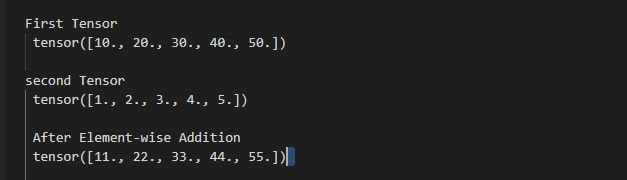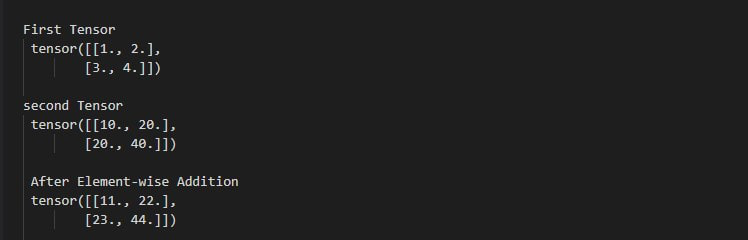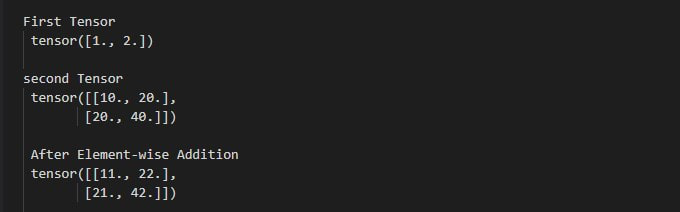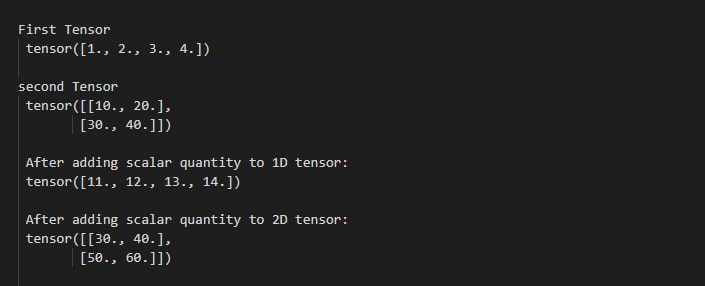# How to perform element-wise addition on tensors in PyTorch?

This function also allows us to perform addition on the same or different dimensions of tensors. If tensors are different in dimensions so it will return the higher dimension tensor.

Parameters:

• inp: This is input tensor.
• c: The value that is to be added to every element of tensor.
• out: This is optional parameter and it is the output tensor.

Return: returns a new modified tensor..

Example 1: The following program is to perform addition on two single dimension tensors.

## Python3

 `# import torch library ` `import` `torch ` ` `  `# define two tensors ` `tens_1 ``=` `torch.Tensor([``10``, ``20``, ``30``, ``40``, ``50``]) ` `tens_2 ``=` `torch.Tensor([``1``, ``2``, ``3``, ``4``, ``5``]) ` ` `  `# display tensors ` `print``(``"\nFirst Tensor \n"``, tens_1) ` `print``(``"\nsecond Tensor \n"``, tens_2) ` ` `  `# Add both the tensor ` `tens ``=` `torch.add(tens_1, tens_2) ` `print``(``"\n After Element-wise Addition\n"``, tens) `

Output:Example 2: The following program is to perform elements-wise addition on 2D tensors.

## Python3

 `# import the torch library ` `import` `torch ` ` `  `# define 2D tensors ` `tens_1 ``=` `torch.Tensor([[``1``, ``2``], [``3``, ``4``]]) ` `tens_2 ``=` `torch.Tensor([[``10``, ``20``], [``20``, ``40``]]) ` ` `  `# display these tensors ` `print``(``"First Tensor \n"``, tens_1) ` `print``(``"second Tensor \n"``, tens_2) ` ` `  `# perform addition on these tensors ` `tens ``=` `torch.add(tens_1, tens_2) ` ` `  `# display final result ` `print``(``"\n After Element-wise Addition\n"``, tens) `

Output:Example 3: The following program is to shows how to perform elements-wise addition on two different dimension tensors.

## Python3

 `# import torch library ` `import` `torch ` ` `  `# define 1D tensor ` `tens_1 ``=` `torch.Tensor([``1``, ``2``]) ` ` `  `# define 2D tensor ` `tens_2 ``=` `torch.Tensor([[``10``, ``20``], [``20``, ``40``]]) ` ` `  `# display tensors ` `print``(``"\nFirst Tensor \n"``, tens_1) ` `print``(``"\nsecond Tensor \n"``, tens_2) ` ` `  `# perform addition on tensors ` `tens ``=` `torch.add(tens_1, tens_2) ` ` `  `# display final output ` `print``(``"\n After Element-wise Addition\n"``, tens) `

Output:Example 4: The following program is to know how to add a scalar quantity to a tensor.

## Python3

 `# Python program to perform element-wise Addition ` `# import the required library ` `import` `torch ` ` `  `# define a tensor ` `tens_1 ``=` `torch.Tensor([``1``, ``2``, ``3``, ``4``]) ` ` `  `# define 2D tensor ` `tens_2 ``=` `torch.Tensor([[``10``, ``20``], [``30``, ``40``]]) ` ` `  `# display tensors ` `print``(``"\nFirst Tensor \n"``, tens_1) ` `print``(``"second Tensor \n"``, tens_2) ` ` `  `# perform addition on 1D tensors ` `t1 ``=` `torch.add(tens_1, ``10``) ` `# display final output ` `print``(``"\n After adding scalar quantity to 1D tensor: \n"``, t1) ` ` `  `# perform addition on 2D tensors ` `t2 ``=` `torch.add(tens_2, ``20``) ` `# display final output ` `print``(``"\n After adding scalar quantity to 2D tensor: \n"``, t2) `

Output:Whether you're preparing for your first job interview or aiming to upskill in this ever-evolving tech landscape, GeeksforGeeks Courses are your key to success. We provide top-quality content at affordable prices, all geared towards accelerating your growth in a time-bound manner. Join the millions we've already empowered, and we're here to do the same for you. Don't miss out - check it out now!

Previous
Next